# Statistics - INPUT - Analysis Parameters

## Teradata Warehouse Miner User Guide - Volume 1Introduction and Profiling

brand
Software
prodname
vrm_release
5.4.4
category
User Guide
featnum
B035-2300-077K
1. On the Statistics dialog box, click on INPUT.
2. Click on analysis parameters.
Statistics > Input > Analysis Parameters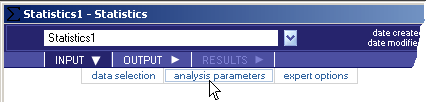The resulting screen has the following options available:
• Basic Statistics Options — The following basic univariate statistics are individually selectable for the analysis. By default, the Number of Values, Minimum Value, Maximum Value, Mean Value and Standard Deviation are selected (and must be selected for graphs to be available). The Check All and Clear All buttons can be used to enable or disable all options.
• Number of Values (required for graphs) — A count of the total number of rows (observations) with values for the specified column.
• Minimum Value (required for graphs) — The smallest value taken on by the column: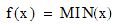• Maximum Value (required for graphs) — The largest value taken on by the column: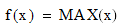• Mean Value (required for graphs) — The average value of the column: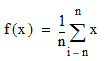where n is the total number of rows (observations) with values for the variable x.

• Standard Deviation (required for graphs) — The standard deviation of the variable. The standard deviation is a measure of how widely values are dispersed from the average value (the mean), and is calculated as follows, based on the entire population (by default):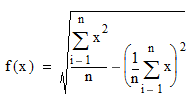If Sample Statistics are chosen, the following formula is used: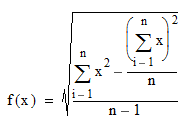In both cases, n is the total number of rows (observations) with values for the variable x.

• Skewness — The skewness of the variable is a characterization of the degree of asymmetry of a distribution around its mean. Positive skewness indicates a distribution with an asymmetric tail extending toward more positive values. Negative skewness indicates a distribution with an asymmetric tail extending toward more negative values.
The measures for Skewness (and Kurtosis) that are provided by Teradata Warehouse Miner are also known as the “Fisher g statistics,” related to the “momental skewness and kurtosis” [D’Agostino, Belanger, and D’Agostino Jr.].
Skewness is calculated as follows, based on the entire population (by default):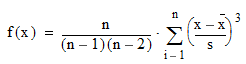If Sample Statistics are chosen, the sample standard deviation, as shown above, is used for s. Otherwise, the population standard deviation is used.

In the above equation, n is the total number of rows (observations) with values for the variable x. Note that skewness is undefined when either the standard deviation of the variable is equal to 0, or the number of occurrences is less than 3.

• Kurtosis — The kurtosis of the variable is a characterization of the relative peakedness or flatness of a distribution compared with the normal distribution. Positive kurtosis indicates a relatively peaked distribution. Negative kurtosis indicates a relatively flat distribution.
The measures for Kurtosis (and Skewness) that are provided by Teradata Warehouse Miner are also known as the “Fisher g statistics,” related to the “momental skewness and kurtosis” [D’Agostino, Belanger, and D’Agostino Jr.].

Kurtosis is calculated as follows, based on the entire population (by default):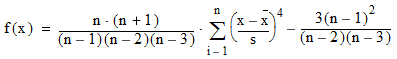If Sample Statistics are chosen, the sample standard deviation, as shown above, is used for s. Otherwise, the population standard deviation is used.

In the equation above, n is the total number of rows (observations) with values for the variable x. Note that kurtosis is undefined when either the standard deviation of the variable is equal to 0, or the number of occurrences is less than 4.

• Standard Error — The standard error of the variable, calculated as the standard deviation divided by the square root of the number of occurrences. Standard error is calculated as follows, based on the entire population (by default):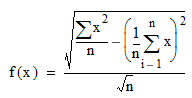If Sample Statistics are chosen, the following formula is used: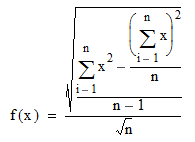In both cases, n is the total number of rows (observations) with values for the variable x.

• Coefficient of Variance — The coefficient of variance of the variable, calculated as 100 times the standard deviation divided by the mean. Coefficient of variance is calculated as follows, based on the entire population (by default):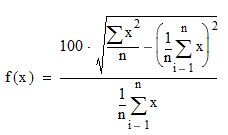If Sample Statistics are chosen, the following formula is used: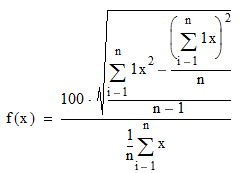In both cases, n is the total number of rows (observations) with values for the variable x. Note that coefficient of variance is undefined when the average of the variable is 0.

• Variance — The variance of the variable, calculated as the square of the standard deviation. Variance is calculated as follows, based on the entire population (by default):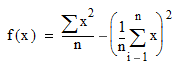If Sample Statistics are chosen, the following formula is used: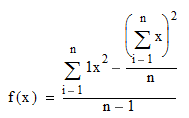In both cases, n is the total number of rows (observations) with values for the variable x.

• Sum — The sum of the variable, calculated as: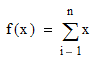where n is the total number of occurrences of this variable.

• Uncorrected Sums of squares — The uncorrected sums of squares of the variable, calculated as: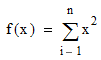where n is the total number of occurrences of this variable.

• Corrected Sums of squares — The corrected sums of squares of the variable, calculated as: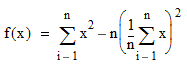where n is the total number of occurrences of this variable.

• Number Select List Items
• Auto-Calculate — When checked, an attempt is made to determine the number of select list items that should be included in the SQL for the Basic Statistics Options. In some cases however, the SQL may fail due to too many select list items being generated, dependent on the number of input columns and the Basic Statistics Options requested. In this case the Auto-Calculate option should be unchecked and a value provided in the Maximum Number... text box below it.
Tip: When processing more than 300 input columns with the first five basic statistics requested, try setting the maximum items to 1000 or less in the text box below.
• Maximum — An integer greater than 0 representing the maximum number of items that will appear in any given SELECT statement generated for the Basic Statistics Options.
• Extended Statistics Options: — The following additional statistics are individually selectable for the analysis. By default, none are selected. The Check All and Clear All buttons can be used to enable or disable all options.
• Values — Extend the Statistical analysis by adding the count of various kinds for the selected column(s), including:
1. Number of Rows
2. Rows with Non-NULL Values
3. Rows with NULL Values
4. Unique Values
5. Rows with Value ‘0’
6. Rows with a Positive Value
7. Rows with a Negative Value
8. Rows Containing Blank Values
• Modes — Extend the Statistical analysis by adding the calculation of Modal or most frequently occurring values.
• Quantiles — Extend the Statistical analysis by adding the calculation of the bottom and top ten percentiles, deciles, quartiles and tertiles.
• Rank — Extend the Statistical analysis by adding the bottom five and top five values and their respective counts.
• Statistical Method
• Sample — Use sample statistics for those statistical calculations where a Sample formula was given.
• Population — Use population statistics for the statistical calculations.